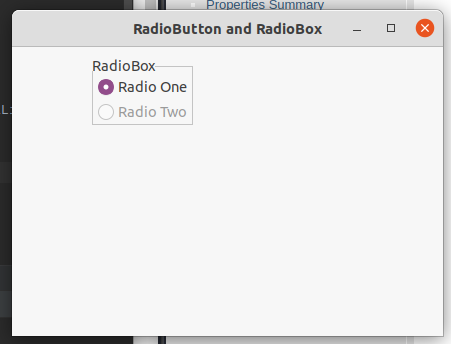Skip to content
Related Articles
wxPython – GetCount() function in wx.RadioBox
• Last Updated : 30 Jun, 2020

In this article we are going to learn about GetCount() function associated with wx.RadioBox class of wxPython. GetColumnCount() function is used to simply return the number of items in the control. No parameters are required by GetCount() function.

Syntax: wx.RadioBox.GetCount(self)

Parameters: No parameters are required by GetCount() function.

Return Type:
int

Code Example:

 `import` `wx`` ` ` ` `class` `FrameUI(wx.Frame):`` ` `    ``def` `__init__(``self``, parent, title):``        ``super``(FrameUI, ``self``).__init__(parent, title ``=` `title, size ``=``(``300``, ``200``))`` ` `        ``# function for in-frame components``        ``self``.InitUI()`` ` `    ``def` `InitUI(``self``):``        ``# parent panel for radio box``        ``pnl ``=` `wx.Panel(``self``)`` ` `        ``# list of choices``        ``lblList ``=` `[``'Radio One'``, ``'Radio Two'``]`` ` `        ``# create radio boc containing above list``        ``self``.rbox ``=` `wx.RadioBox(pnl, label ``=``'RadioBox'``, pos ``=``(``80``, ``10``), choices ``=` `lblList,``                                         ``majorDimension ``=` `1``, style ``=` `wx.RA_SPECIFY_COLS)`` ` `        ``# print total items in radio box``        ``print` `(``self``.rbox.GetCount())`` ` ` ` `        ``# set frame in centre``        ``self``.Centre()``        ``# set size of frame``        ``self``.SetSize((``400``, ``250``))``        ``# show output frame``        ``self``.Show(``True``)`` ` ` ` `# wx App instance``ex ``=` `wx.App()``# Example instance``FrameUI(``None``, ``'RadioButton and RadioBox'``)``ex.MainLoop()`

Console Output:

```2
```

Output Window:Attention geek! Strengthen your foundations with the Python Programming Foundation Course and learn the basics.

To begin with, your interview preparations Enhance your Data Structures concepts with the Python DS Course. And to begin with your Machine Learning Journey, join the Machine Learning – Basic Level Course

My Personal Notes arrow_drop_up